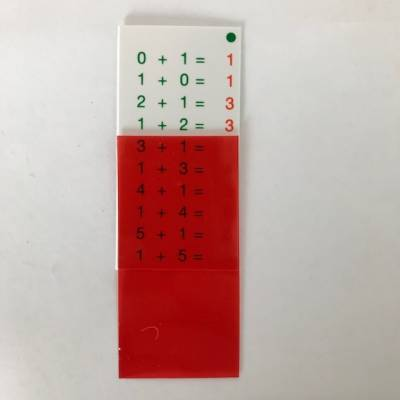JP-Numeracy
R 184JP-Numeracy
Division Buddy Cards
R 184 R 159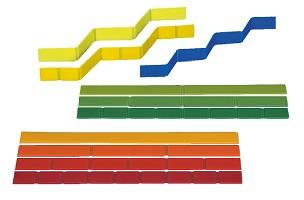JP-Numeracy
Fraction Set Linear
R 338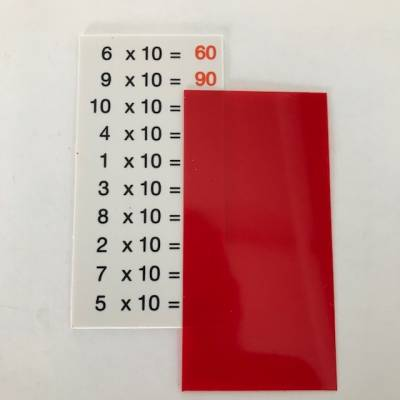JP-Numeracy
Multiplication Buddy Cards
R 184 R 159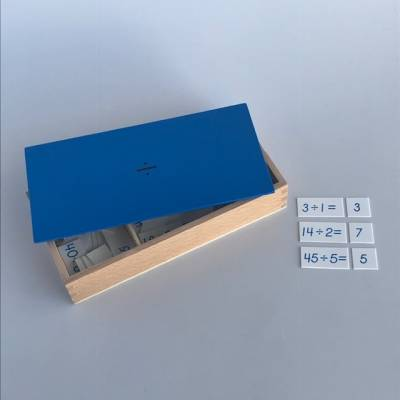CH - Mathematics
Premium Divisions Equations & Dividends Box
R 629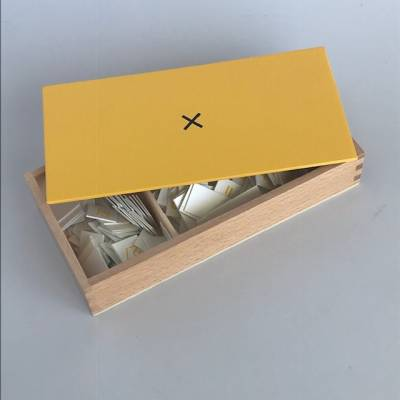CH - Mathematics
Premium Multiplication Equations and Products Box
R 629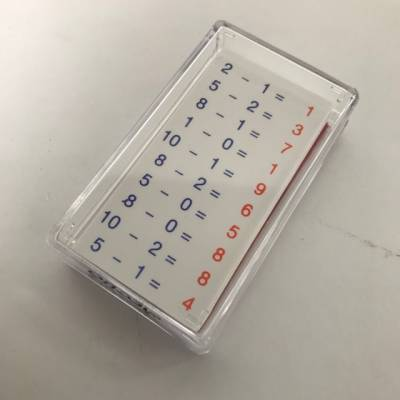JP-Numeracy
Subtraction Buddy Cards
R 184 R 159